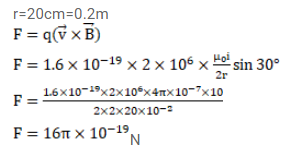# A circular loop of radius 20cm carries a current of 10A.

Question:

A circular loop of radius $20 \mathrm{~cm}$ carries a current of $10 \mathrm{~A}$. An electron crosses the plane of the loop with a speed of $2.0 \times 10^{6} \mathrm{~m} / \mathrm{s}$. The direction of motion makes an angle of $30^{\circ}$ with the axis of the circle and passes through its center. Find the magnitude of the magnetic force on the electron at the instant it crosses the plane.

Solution: